In the last section, we set a couple of problems, and asked you to devise a program to come up with the answer.

1. Write a program to add up the integers from 1 to 10, and put the result in to the memory location at offset zero.
2. Alter the program to add up the integers from 1 to 15 and put the result in to the memory location at offset zero.
3. Alter the program again, to add up the numbers from 5 to 15, and put the result into the memory location at offset zero.

If you wrote a program for problem (A) which ended up with the number 55 in memory location zero, then congratulations - you have not only written your first program solo, but you've also devised your first algorithm: a description of a process required to take some input and produce a desired result.

In this section, we'll look at some programs I've devised to solve these 3 related problems. Your program may not look exactly like any of mine, and if you got the right number in the right place, then that's great. There's no 100% right answer - you're always making compromises and improvements. And even if you did get the right answer, it is always interesting to look at other people's solutions, and see if there's something you can learn from them.

We're going to evolve our solution from a very simple-minded approach, to a more sophisticated algorithm, and look at the implications and compromises we're making along the way.

So, here's a first stab at an algorithm for solving problem (A). It is not a very complicated one: we could do the addition one line at a time, long-hand.

``````load r1 0
write 0 r1
exit
``````

(Notice that we remember to set the accumulated total to zero before we start - we don't know what might have been in the register before we began.)

That's a pretty decent stab at it. It gives us the right answer, and only takes 12 instructions to do it.

When we go to problem (B), it starts to look a bit less great. I got a bit bored typing this in, even with the help of copy and paste.

``````load r1 0
write 0 r1
exit
``````

Imagine we wanted to add the whole sequence for 1…10,000! That'd be a lot of instructions. 10,003 to be precise! In fact, I don't think we've got enough program instruction memory in this toy computer to do that. Also, don't forget that every instruction we execute takes a little bit of time. If we're running in the public cloud that directly costs us a little bit of money (or indirectly via our electricity bill if we're running at home or in the office, in the form of the little bit of energy we expended in the execution of each instruction). So, ideally we'd also like to execute as few as possible.

It turns out that these are often competing constraints.

Let's look at the first part of this challenge. First - can we make our program more compact, such that we could reasonably sum integers up to 10,000 or more? To do that, we need to understand both the problem, and the algorithm we are using to solve it. Let's start by describing it more precisely.

## Describing our algorithm - flowcharts

There are lots of ways of describing an algorithm - we can do it in code, in everyday language, mathematically, or graphically, for example.

Instead of using more words, let's look at a graphical representation of our first algorithm, called a Flow Chart.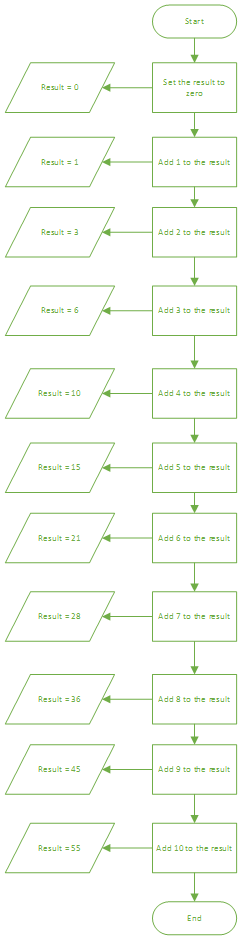Long, isn't it, but quite expressive?

We're using four symbols in this diagram.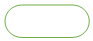The start or end of the algorithm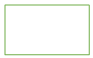A process or operation to be executed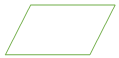Some data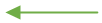The flow of control through the algorithm (hence 'flow chart')

It gives a nice, clear, step-by-step view of what we are doing. Notice that many of these steps are the same. If we were trying to explain this to someone in ordinary language, we might say

To start, set the result to zero.

Then, add 1 to the result.

Then, add 2 to the result.

(… and keep doing that sort of thing until …)

Then, add 9 to the result.

Then, add 10 to the result.

Then, you are done.

Anybody with some really basic maths would understand what you meant. But, unfortunately, computers are (in general) a bit slow on the uptake, and need everything explained to them in precise detail. How could we leave out those steps where we've used a hand-wavy description to avoid repeating ourselves, and yet still describe precisely what needs to be done?

One possible way is to use a loop.

## Loops (power and pitfalls)

Let's re-describe that process in ordinary language, and find some way of explaining the boring repetitive bit, such that we don't just wave our hands and skip over it. Here's my attempt.

To start, set the result to zero.

Then set the current number to 1

Then add the current number to the result

Then add 1 to the current number to give the next number

If the current number is less than or equal to 10 then go back and repeat the previous two steps, otherwise you are done.

If we want to be really precise, we could write this down again in language somewhere between an actual program and ordinary language. We call it pseudocode. Given the low-level language you've been dealing with up to now, this should be fairly easy for you to interpret - and that's the idea. It isn't any particular programming language, but it is just as precise as any programming language, so more or less any programmer should be able to understand it. We're also being meticulous about describing what the algorithm is supposed to do, what input it needs, and how to interpret the result.

Description: Calculate the sum of a particular sequence of consecutive positive integers

Input: The first number of the sequence,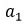and the last number of the sequence an

Output: The sum of the integers between a1 and an(inclusive)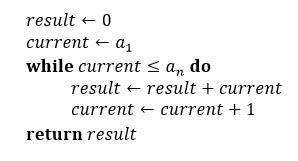Notice we're using a mixture of 'ordinary language' and mathematical symbols to describe precisely what our algorithm does.

There are a couple of terms that you might need help with: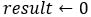as "result changes to 0". The arrow is just a shorthand for a phrase like "changes to" or "becomes".

Then there's: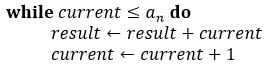You can read this as "while the current value is less than or equal to the last number in the sequence, keep doing the steps in the list below". Notice that we've used indentation to make it easy to see where that list of steps starts and ends. Those steps can be read as "the result changes to the value of result plus the current value", and "the current value change to the current value plus 1".

This pattern, where you go back and repeat a previous step based on some condition is very common in imperative programming, and we call it a loop. (This specific example is called a while loop for obvious reasons.) You can see why we call it a loop if we express this program as a flow chart.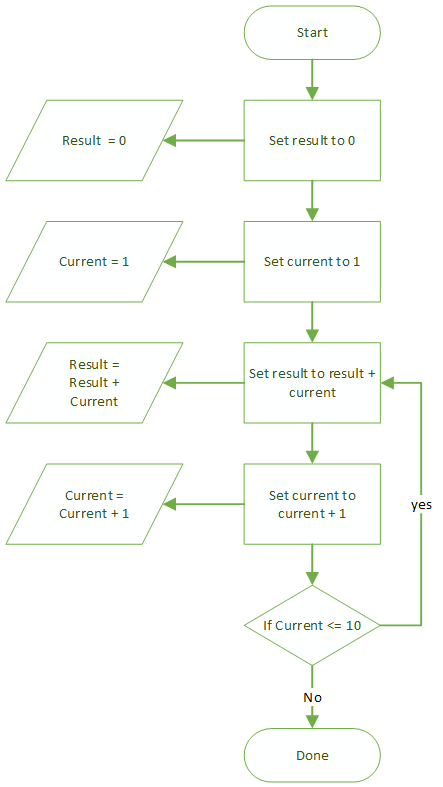The first thing to notice is that we've added an extra symbol to our set, the diamond for a decision:The start or end of the algorithmA process or operation to be executedSome dataThe flow of control through the algorithm (hence 'flow chart')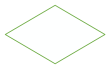Decision

The diamond has 2 arrows coming out of it, one labelled 'yes' for the path to take if the statement in it is true. The other labelled 'no' for the path to take if the statement is false. Notice how the 'yes' arrow 'loops back' up the diagram from the decision point, to repeat a part of the process.

Let's restructure the program to implement this new, looping version of the algorithm. We're going to make use of registers to remember the minimum and maximum values of our sum, and take advantage of our `COMPARE `and `JUMPLTE `instructions to minimize the amount of repetitive code we have to write.

This program uses `r0 `to store the current number to be added, `r2 `to store the maximum number to be added, and `r1 `to accumulate the result. I've numbered the lines to make it easier to calculate the offset when we jump, and added some details comments. (You should leave both of these bits out if you edit your program and run it in our model computer.)

1. `load r2 10 ; We want to add up the numbers up to 10`
2. `load r0 1 ; We want to start with 1`
3. `add r1 r0 ; add the current number in r0 to the total in r1`
4. `add r0 1 ; increment the current number by 1`
5. `compare r0 r2 ; compare the current number with the maximum number `
6. `jumplte -3 ; if the current number is less than or equal to the maximum number, jump up 3`
7. `write 0 r1 ; otherwise, write the accumulated total from r1 into memory location at offset 0`
8. `exit`

The critical bit of code in this program that implements the loop is this:

``````add r1 r0
compare r0 r2
jumplte -3
write 0 r1
``````

Here's how it works.

Once we've added the current number stored in `r0` to our accumulated total in `r1`, we increment ("add 1 to") `r0` so that it contains the next number to add. `r2` still contains the largest number we are interested in.

So, if the current number (`r0`) is less-than-or-equal-to the maximum number (`r2`), then we go back up 3 instructions. This takes us back to the line that adds the current number to the total. We keep going round and round like this, each time jumping back to the `add `instruction.

What happens when the current number is greater than the maximum number? We've seen before that in that case the `JUMPLTE `instruction does nothing, so we will step on and write the result into memory and exit.

Let's redraw the flowchart, with our actual instructions in the boxes so you can see how precisely similar they are.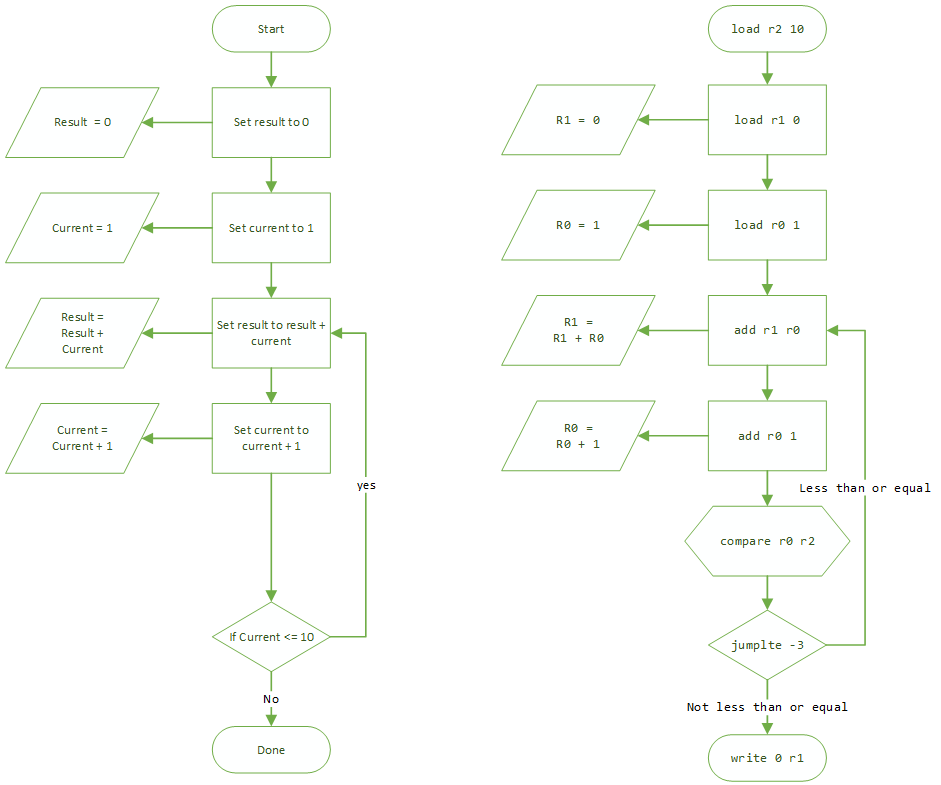(We've added a hexagonal symbol on the diagram, which we use to represent steps that are purely a preparation for a comparison.)

Now that we've re-written the algorithm like this, it is easy to adapt it to solve problems (B) and (C) from the previous exercise.

For problem (B), to change the range of numbers to add from 1 to 15, we simply increase the value stored in `r2`

``````load r2 15 ; We want to add up the numbers up to 15
load r1 0 ; Set the accumulated total to zero
add r1 r0 ; add the current number in r0 to the total in r1
add r0 1 ; increment the current number by 1
compare r0 r2 ; compare the current number with the maximum number
jumplte -3 ; if the current number is less than or equal to the maximum number, jump up 3
write 0 r1 ; otherwise, write the accumulated total from r1 into memory location at offset 0
exit
``````

For problem (C), to change the start of the range, we change the value stored in `r0`:

``````load r2 15 ; We want to add up the numbers up to 15
load r1 0 ; Set the accumulated total to zero
add r1 r0 ; add the current number in r0 to the total in r1
add r0 1 ; increment the current number by 1
compare r0 r2 ; compare the current number with the maximum number
jumplte -3 ; if the current number is less than or equal to the maximum number, jump up 3
write 0 r1 ; otherwise, write the accumulated total from r1 into memory location at offset 0
exit
``````

That's great - we could imagine increasing the total of numbers in the sequence to any arbitrarily large number, and we wouldn't run out of program memory.

Let's remind ourselves of the original program:

``````load r1 0
write 0 r1
exit
``````

This needed as many instructions as there were numbers to add, plus a few instructions to set up and finish. We say that the number of instructions in the program instructions memory scales linearly with the number of items to process, or is of order n (where 'n' is the number of items to be processed). We have a shorthand notation for this called big O notation: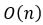In general, we estimate the order of an algorithm by finding the bits that grow the fastest as we add more items into the input.

We could represent the storage cost of our algorithm diagrammatically, drawing a box scaled to the number of instructions required to implement each part of the algorithm.Now, imagine that we double the number of items in the sequence and sketch it again (reducing the scale by half so it fits on the page)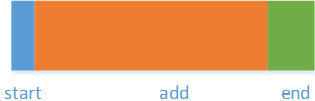And double the number of items again, reducing the scale by half once more.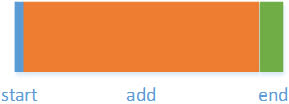Each time, the central "add" section becomes a more and more significant part of the storage cost of the algorithm.

Now, imagine a huge number of items. The contribution of the start and finish become vanishingly small, and the budget is taken up (to all intents and purposes) entirely by the central piece of the work, so we can ignore them when we estimate the order of our algorithm.

So, back to our program. With the new version, no matter how big the number, we only need a program 9 instructions long. So, we've really optimized our program storage. The storage requirement of this program is constant, or of order 1: O(1).

(We will look at how to calculate this more formally later on.)

This is clearly a good thing. But, if you look closely, you'll realise we're actually executing a lot more instructions than we were before. To add each number in the original program, here's the code we add to execute:

`add r1 3`

But to add each number in the new program, here's the code we have to execute:

``````add r1 r0
compare r0 r2
jumplte -3
``````

Four instructions in the new program for each instruction in the old program. So the new program is more computationally expensive. About four times more, in fact, if you ignore the few instructions they both have for setup and completion, and you assume that all those instructions take the same amount of time to execute (which they almost certainly don't – that `jumplte` is probably more effort than an add).

This may (or may not) be significant to us.

If we think about the computational cost of both implementations, they scale linearly with the number of items in the collection, so both the old and the new algorithm are O(n), but the new one is more expensive - especially for just a few items in the sequence. It could be cheaper to write the instructions out individually if we have less than, say, 4 numbers in the sequence, than it is to use the loop.

We call this process of trying to find a better, cheaper way to write our algorithms optimization. In this case, we've optimized our program for program storage, at the expense of some speed. We've made a significant improvement in the former (going from O(n) to O(1)), while still maintaining our O(n) computation cost – albeit that the new algorithm is potentially 4 times slower.

You will often find that you are making these trade offs - space for time, or vice versa. Sometimes, you discover that an optimization for space actually improves the time as well - if a significant portion of the time was taken allocating or deallocating resources.

## Optimization (premature & otherwise)

There's another point to make about optimization, though. I'm using a lot of contingent words like 'could' and 'may or may not' in terms of the computational cost or benefit of one algorithm or another. This is because we haven't measured it. And while it is almost certainly the case that an order-of-magnitude improvement in the efficiency of an algorithm in either storage or computation (or both) will improve the overall performance of your software, that is by no means guaranteed, especially where there are complex interactions with other parts of the system, and different usage patterns. We could expend a week's effort trying to improve this algorithm, get it as good as it could possibly be, and yet it would still not give us any real benefit, because the end users only cause the code to be executed once a month, it completes in 50ms, and that happens overnight as part of a job that takes 20 hours to run. Worse still, we didn't measure how long it took in a variety of real-life situations to provide a baseline, and then repeat those measurements to see if our so-called "optimized" version has actually had a positive effect

One of the things programmers, in general, are very bad at doing, is optimizing irrelevant things. We call this premature optimization. We also tend to focus on the optimization of little details. We call this micro-optimization. It is very tempting to get drawn into this as we have to be so focused on the minutiae of the code we are writing, that we can lose the big picture. Our optimizations can also make the code harder to read, and more difficult to maintain - and perhaps also more prone to bugs.

You could argue that both the programs we've written so far implement the same basic algorithm - they step through each item in the sequence in turn, adding it to the total. The second version uses a loop to do that. The first version just expanded it so that there is an instruction that represents each iteration around the loop (we call that loop unrolling).

If we're going to significantly improve both the storage requirements and the computational cost of this program, we're going to need an algorithm that is O(1) for both storage and computation. Algorithm selection almost always has a bigger impact on performance than micro-optimizations. So, can we come up with a better one?

## Looking for a better algorithm

Let's look at the sum of the numbers from 1 to 15 again.

We can write this as (leaving out some of the terms in the middle to avoid getting too bored…)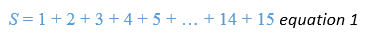Equally we could start at the other end, and write it as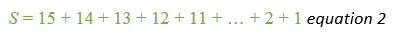We get exactly the same answer.

Now we're going to do a bit of simple algebra - we're going to add these two equations together. In this case, the equations are so simple that we can do that by adding up all the terms on the left hand sides, and all the terms on the right hand sides. I've coloured them according to the original equations so you can see where the terms came from.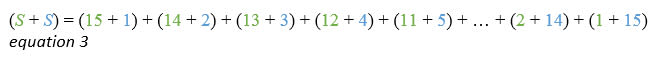Let's look at the right hand side of equation 3 more closely. For each of the 15 terms in our original sum, we've still got exactly one term - and it's an addition. Moreover, that sum is always the same - in this case 16. In fact, we've got 15 lots of 16.

Rewriting that again, then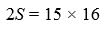And dividing through by 2: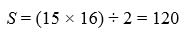Which looks very much like the right answer.

Before we delve into this further, let's try that again for the case when we're adding up the numbers from 5 to 15.

There are 11 numbers between 5 and 15 (inclusive). First, we'll write them out from low to high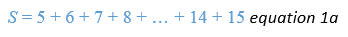And then from high to low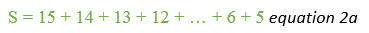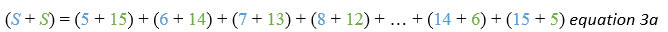Again, we have one term on the right hand side of equation 3a for each of the 11 terms we had in the original sum, and this time each of those terms adds up to 20.

So,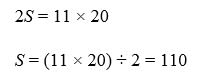Right again.

Now, let's think about the general case of some starting integer m to some general maximum integer n.

First, how do we calculate the number of terms in this case?

Well, in the case of the sequence 1…15, there are 15 terms, which is (15 - 1) + 1.

For the sequence 5…15 there are 11 terms, which is (15 - 5) + 1.

In the general case, there are (n - m) + 1 terms, i.e.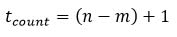Another way of looking at the last term 'n' is to say that it is the starting term, plus the number of terms, less one (to account for the fact that we've already included the first term.) i.e.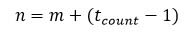From our previous equation, we can see that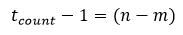So, what does our sum look like if we write it out in these general terms?

Here's the version starting with the first number in the sequence, m.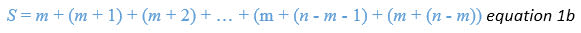Notice how we've expanded the last few terms to calculate them using our expression for the number of items in the sequence. This looks a bit redundant: after all, m + (n-m-1) = n-1 and m + (n-m) = n, but you'll see why it is important we express it that way in a moment.

As before, we'll write that the other way around, high to low, expressing it in terms of the highest number in the sequence, and our expression for the number of terms.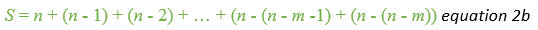And then add them up again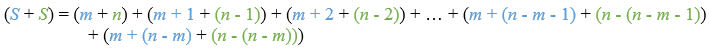Here, you can quickly see that each term adds up to (m + n) - which is suggestive of a solution. (Note that this isn't a proper proof! Anything could be happening in those bits in the middle. But, as it happens, this solution is correct, and generalizable.)

So, just as before, we can write our equation as

2S = (number of terms) * (value of each term) , or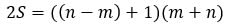If we divide both sides by 2, we have a general formula for calculating the sum of all the integers from m to n: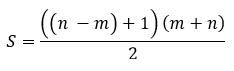Or we could use the mathematical sigma notation - it means the same thing: "the sum of each i from i = m to i = n".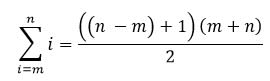Armed with this new algorithm, we can write a much better program to calculate the sum, in terms of both the space it needs, and the number of instructions it has to execute.

### Exercise 1

Can you devise a program to calculate the sum of any sequence of consecutive, positive integers?

Hint: There are instructions in our computer called `mul` and `div` which perform multiplication and division just like our `add `and `sub `instructions.

### Exercise 2

A geometric progression is one in which each term is a constant multiple of the previous term: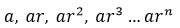For example 3, 6, 12, 24, 48 is a geometric progression where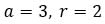The formula for the sum of a geometric progression (which we call a geometric series) where a is the value of the first term in the sequence, and r is the constant multiplier is: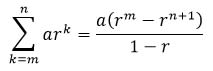Implement a program that can calculate the result of the geometric series for any arbitrary values of a, r, m and n.

Can you estimate the compute cost of your implementation, using big O notation? What about the storage cost?

## Summary

We've learned several important things in this section:

1. Several ways to represent algorithms, in the forms of code, flowcharts, pseudo-code and just ordinary language.
2. How to estimate the order of an algorithm, in terms of both its storage requirements and its computational cost, and how to communicate that using big O notation (e.g. O(1), O(n), O(n^2 ) etc.)
3. We've seen how to go about optimizing our algorithms for either space, storage or both - including the importance of measuring both before and after any change to see if it has had any significant positive effect for the effort we've put in to doing it
4. We've seen the importance of picking the best algorithm for the job, given the kind of input you are working with, and that the selection of a good algorithm can have far more impact on the cost of execution than micro-optimizations of the implementation.

In the next section, we're going to look at the data memory in more detail, and learn how we can represent numbers in a computer.